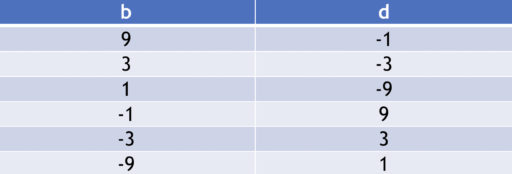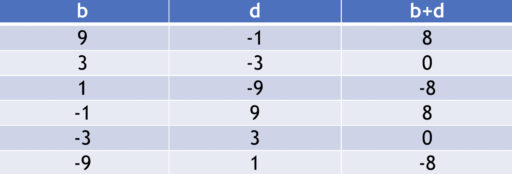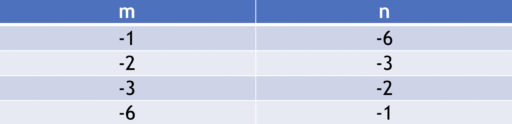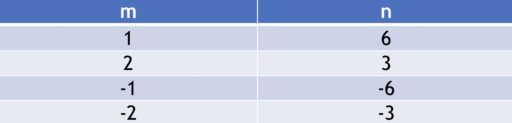In a previous post, we have seen how to use the quadratic formula to solve quadratic equations. Here, we will discuss another method: factoring.

The basic idea behind factoring is the same idea that drives many military campaigns: divide and conquer. A quadratic equation (like) is complicated. Finding its solutions is hard. But if we break it down into its component parts:then it is easy to find its solutions! After all, the only way forto be equal to 0 is iforor both are equal to 0. Otherwise, we would have two non-zero numbers multiplied by each other, which always yields a non-zero number.

And the only values ofthat makeorequal to 0 are -3 and -2. Thus,are the only solutions ofAnd since, we conclude thatare the only solutions ofNow, let’s set out the general steps for factoring a quadratic equation:

Method of Factoring

1. Ensure 0 is on one side of the equation
2. Factor our quadratic expression as a product of two linear expressions
3. Find the-values that make each linear expression equal to 0

Example
Suppose we start with:1. Ensure 0 is on one side of the equation
Remember that we can add/subtract/multiply/divide as we like, provided that we do it to both sides. (Note, though, that we are not allowed to divide byor 0. Dividing byeliminates a solution and dividing by 0 is just prohibited by the rules of arithmetic).Let’s subtractfrom both sides. Then we get:2. Factor our quadratic expression as a product of two linear expressions
Linear expressions are just expressions that have a degree of 1 or lower. For example, things likeandandare all linear expressions.
In the next section, we will further discuss how to find the right linear expressions. Here, we show how we might do that for the quadratic expressionWe know that we are ultimately looking for something like:whereare all constants. This is becauseis the general form for any linear expression. Let’s suppose we have already found the rightThen, multiplying things out, we get:Now, on the left hand side of the equation, we have that the only coefficient ofisOn the right hand side, we know that the coefficient ofis 1. Therefore,(since otherwise, the two sides of the equation would not be equal, since they would have different amounts of).Now, that means we could haveororand many more possibilities. Just to keep things simple, though, let’s try assuming thatand see if that leads us to a solution. (If it does not, we can always revisit this step and try something else).So suppose. Then, we get:Now, we want to find some values ofsuch that:Now, we will generally solve these problems by listing all the possible integers such thatThen, we will look at them and see if any of those combinations will add up to. If we cannot find an integer solution, then your best bet is to give up on this method and just use the quadratic formula.
Here are all the possibilities forYou can construct such a list by going through the factors of 9. For every factor of 9 (e.g. 1) there is some integer (in this case, 9) so that when they are multiplied together, they equal 9 (). And to deal with the negative sign, simply alternate which of the two factors (whether 1 or 9) is negative.Running through the table, we get:Thus there are two possibilities:orIt doesn’t matter which one you choose. Let’s pick the first one.
Then, by plugging in our values for, we getwhich is exactly what we wanted.

3. Find the x-values that make each linear expression equal to 0
Then,implies that. AndimpliesThus,Those are the solutions toFactoring into Linear Expressions

The trickiest step when using factoring to solve a quadratic equation is finding the linear expressions to multiply together. Here, we will only deal with quadratic equations of the form:whereare constants. In other words, we assume that the coefficient ofis 1.

Now you can factor equations that have other coefficients forFor example,can be factored as, thereby yielding the solutions. But dealing with these cases is harder, so you are better off, generally, just applying the quadratic formula.

Here are the steps for factoring our quadratic equation into linear expressions:

1. Write out the factors of our constant,2. Write out the pairs of factors that multiply together to get3. Go through them to see which pairs of factors add up to4. (If time) Check our solution

Let’s look at another example. Suppose we have:We want to factor this into an expression that looks like:whereare integers. Now, let’s follow our steps.

Factoring into Linear Expressions

1. Write out the factors of our constant,has the factors 1, 2, 3, and 6.
2. Write out the pairs of factors that multiply together to getThe easiest way to do this is just to go from least factor to the greatest factor in the first column, and then write down the number needed in the second column so that their product is equal to 6. Here, we have:And don’t forget that combinations with negative numbers also count:Now, this may look like a lot of combinations to try. But note that the first row of each table looks like the fourth row, just with the order of the columns flipped. Since it doesn’t matter which order the numbers are in (since), we don’t need to check both rows. Similarly for the second and third row. Thus, we only need to check:3. Go through them to see which pairs of factors add up toNow, we just check whether any of these combinations add up to -5. And. Thus, we have our answer:.
4. (If time) Check our solution
If you have the time, it is highly recommended that you check your solution since it only takes a second to do. And if your solution is wrong, that’s valuable information. In our case:so our solution is correct.

Practice Problems

Solve the following quadratic equations via factoring:

1.2.3.4.5.